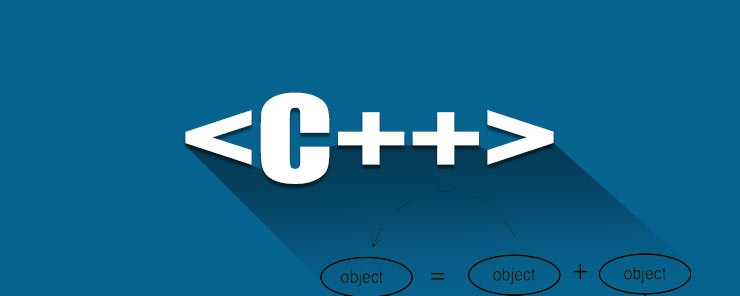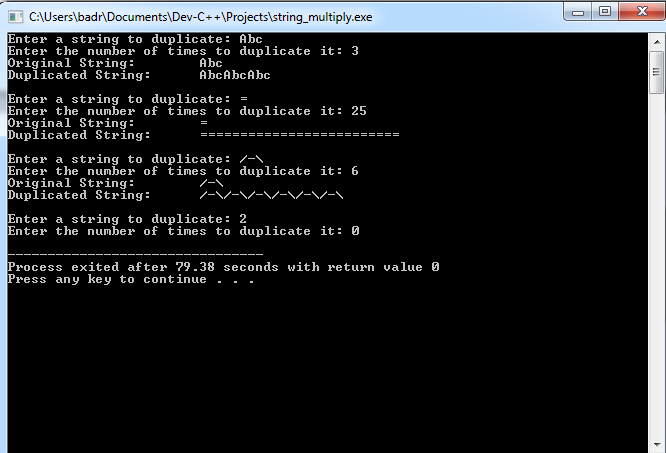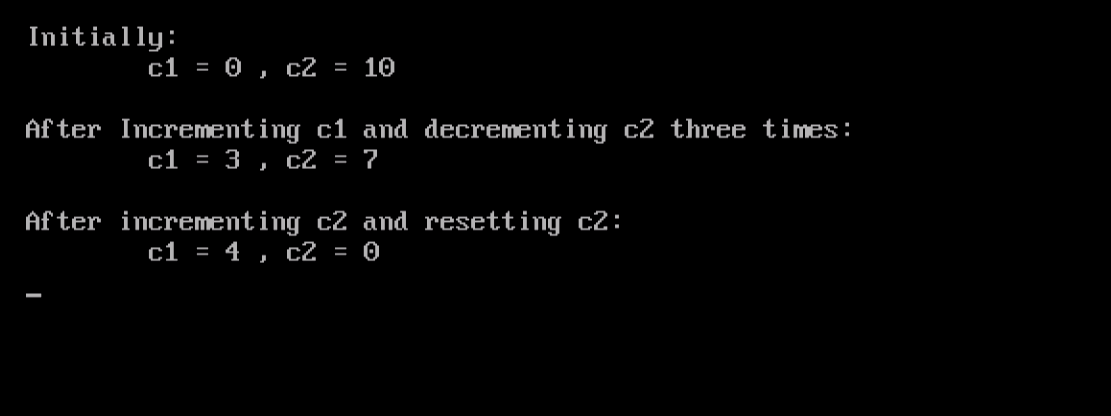Similar to functions, operators can also be overloaded. An operator can also have more than one definition. In the previous article, we have seen how the plus + sign (that does the addition operation in arithmetic) has been re-defined by the string class to do the string concatenation job. This is a great feature of C++ and OOP that allows you to break the limits of the ordinary programming languages (including C), and master the language tools. Our topic for today’s article is operator overloading. Wish you nice reading.

The operator Keyword
To overload an operator, C++ offers a special keyword: the operator keyword.

Syntax

```RETURN_TYPE  operator OPERATOR ([ARGUMENTs])
{
Function Body
}
```

Binary operators require two operands. Well-known examples for these are +, -, *, /, and %. These operators are mainly used in arithmetic operations. However, we could redefine an operator to work with user-defined data types as well. Okay, let’s simplify things using an example.

Example
The string class has overloaded the + operator to do string concatenation. What about overloading another operator to do string repetition (multiplication) operation? The operation requires mainly a string operand (the string that will be duplicated), and an integer operand that represents the number of times to duplicate that string. The result should be assigned to a string object. Interesting challenge, huh?!

Okay, let’s say the operand to overload is the asterisk * sign (to be analogous to its original meaning: multiplication).  Now read the following code:

```#include <iostream>
#include <conio.h>
using namespace std;
string operator * (string str, int x)
{
string S = "";
for(int i=1;i<=x;i++)
S += str;
return S;
}

int main()
{
int c;
string S1,S2;
do
{
cout << "Enter a string to duplicate: ";
cin >> S1;
cout << "Enter the number of times to duplicate it: ";
cin >> c;
if(c<1)
continue;
S2 = S1 * c;
cout << "Original String:\t" << S1 << endl;
cout << "Duplicated String:\t" << S2 << endl << endl;
}
while(c>0);
getch();
return 0;
}
```

Let’s see how this program will behave when executed:Now, let’s explain things:

• Before the main() function, the following line appeared:
`string operator * (string str, int x)`

This line declares the * operator. It tells the compiler that whenever it sees this operator (and realize that it is the intended version by checking the number of arguments and their data types), the compiler should invoke this function.

• Inside the main() function:
`S2 = S1 * c;`

This statement uses the overloaded * operator to duplicate the S1 string c times, and assigns (returns) the result to string S2.

Again, what makes the compiler decide to use this overloaded version, or the other one (used for arithmetic multiplication) is the data types of the operands, which are a string and an integer.

In a similar way, we can overload unary operators, like ++ and —

Example
Back to the counter class example (Article 16 – More on OOP)

```class counter
{
private:
int c;
public:
counter(int val)
{ c = val; }
counter()
{ c = 0; }
int getCount()
{ return c; }
void increment()
{ c++; }
void decrement()
{ c--; }
void reset()
{ c = 0; }
};
```

We need to use the ++ and — operators instead of increment and decrement member functions, respectively.

```void operator ++ ()
{ c++; }

void operator -- ()
{ c--; }
```

Now, the main function that will use it:

```void main()
{
clrscr();
counter c1;
counter c2(10);
cout << "\nInitially:\n\tc1 = " << c1.getCount() << " , c2 = "
<< c2.getCount() << endl;
for(int i=0;i<3;i++)
{ c1++; c2--; }
cout << "\nAfter Incrementing c1 and decrementing c2 three times:\n"
<< "\tc1 = " << c1.getCount() << " , c2 = " << c2.getCount()
<< endl;
c1++; c2.reset();
cout << "\nAfter incrementing c2 and resetting c2:\n"
<< "\tc1 = " << c1.getCount() << " , c2 = " << c2.getCount()
<< endl;
getch();
}
```

Executing the counter2.cpp program will give us the following output:Great!

Summary

In the next article, we are going to start talking about Inheritance; an important and constitutive topic that is bound to be interesting. See you then.

### Organic vs Paid Digital Marketing

21,501Fans
4,209Followers
104,000Subscribers

### 5 Tips and Tricks To Secure Your Website Today

- Advertisement -